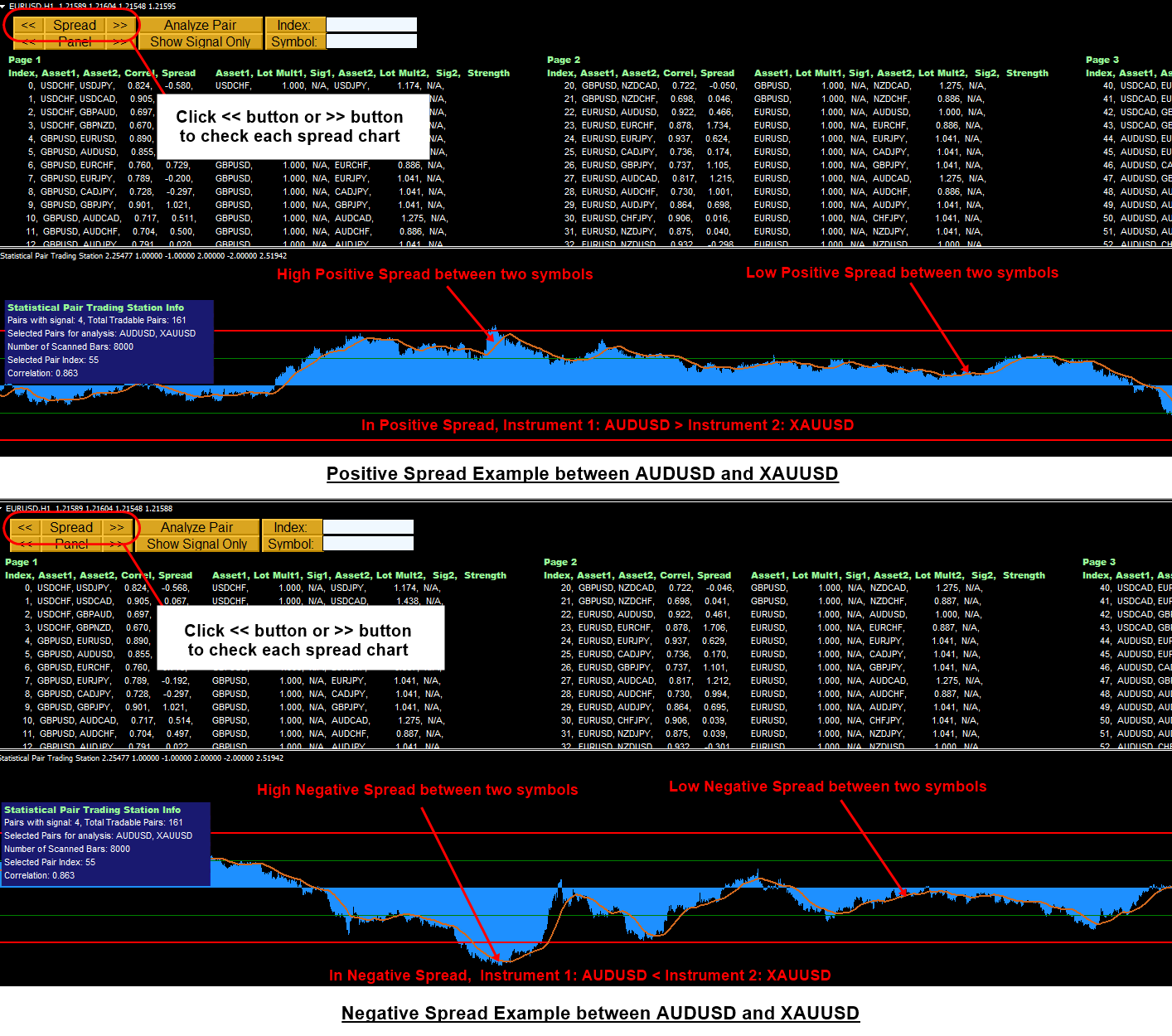From Pairs Trading Station in MetaTrader version, you can access the Spread Chart, which is mathematically processed to show the distance between two financial instruments (or between two assets).

You can access the Spread Chart by clicking << button or >> button  near the Spread button.

Here is the brief guide on how to interpret the Spread Chart. In this example, we use AUDUSD and XAUUSD as asset 1 and asset 2. The absolute magnitude of these two assets are compared in the normalized sense to compare apple to apple and pear to pear.  Two cases exist including the positive spread and negative spread.

In positive spread, AUDUSD (i.e. asset 1) is greater than XAUUSD (i.e. asset 2). Higher the positive spread means that AUDUSD is much greater than XAUUSD in the normalized sense.  If the positive spread is small, then the difference between AUDUSD and XAUUSD is also small.

In negative spread, AUDUSD (i.e. asset 2) is smaller than XAUUSD (i.e. asset 2). Higher the negative spread means that AUDUSD is much smaller than XAUUSD in the normalized sense. If the negative spread is small, then the  difference between AUDUSD and XAUUSD is also small.

Further you can access more detailed trading operation with below Pairs Trading Manual.

https://youtu.be/fAE9pByxZDA